Follow

In this guide we go through the steps in order to grade an ended exam. We will step by step go through the grading process for different question types and also the tools that can be used while grading an exam.

After you have ended an exam you can now start grading. You can find your ungraded exams under the tab Ungraded (see figure 1).Figure 1

Find the exam you want to grade.  Select the green Grade button. (see figure 2)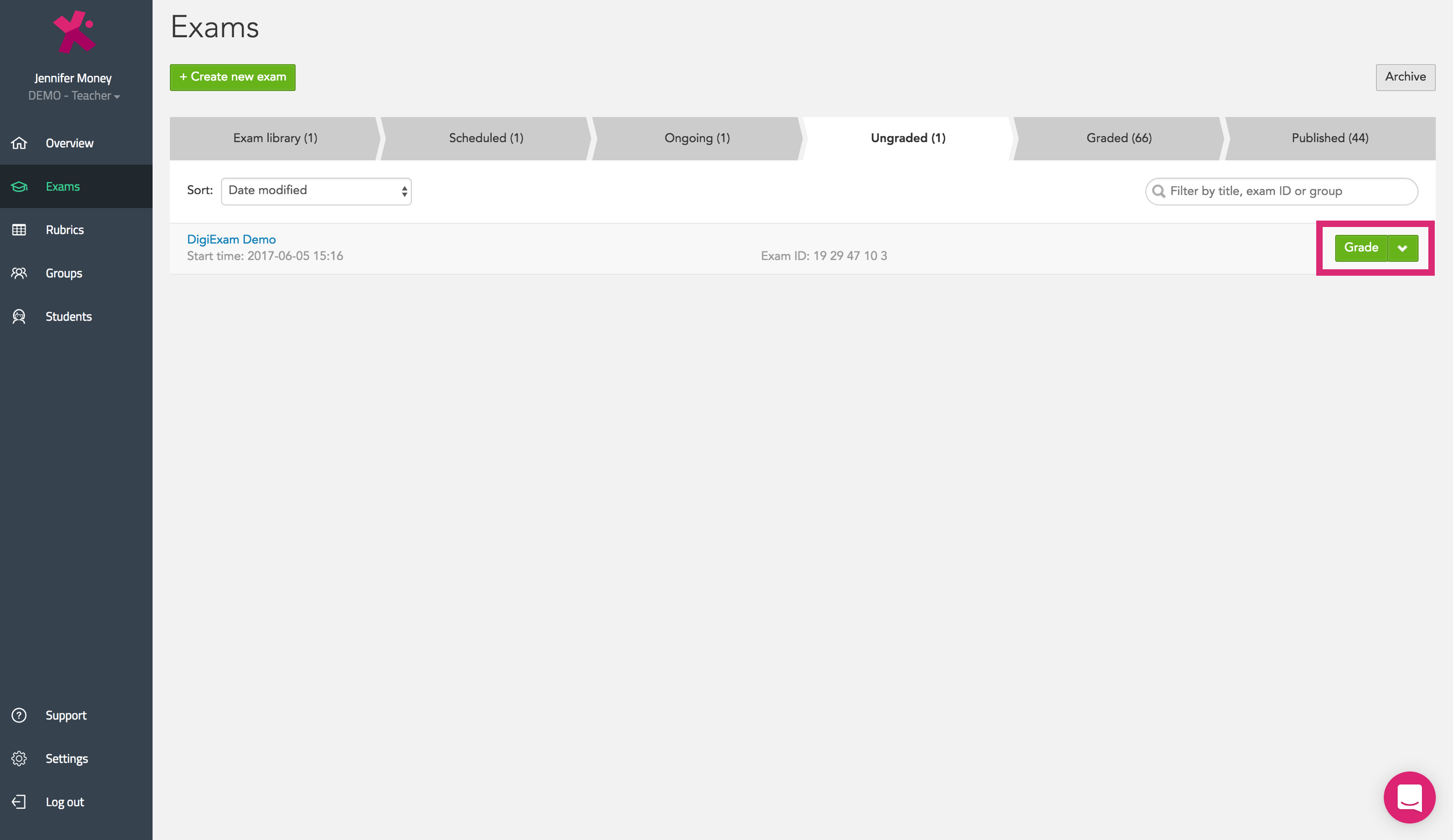Figure 2

Select the green Continue grading button. (see figure 3)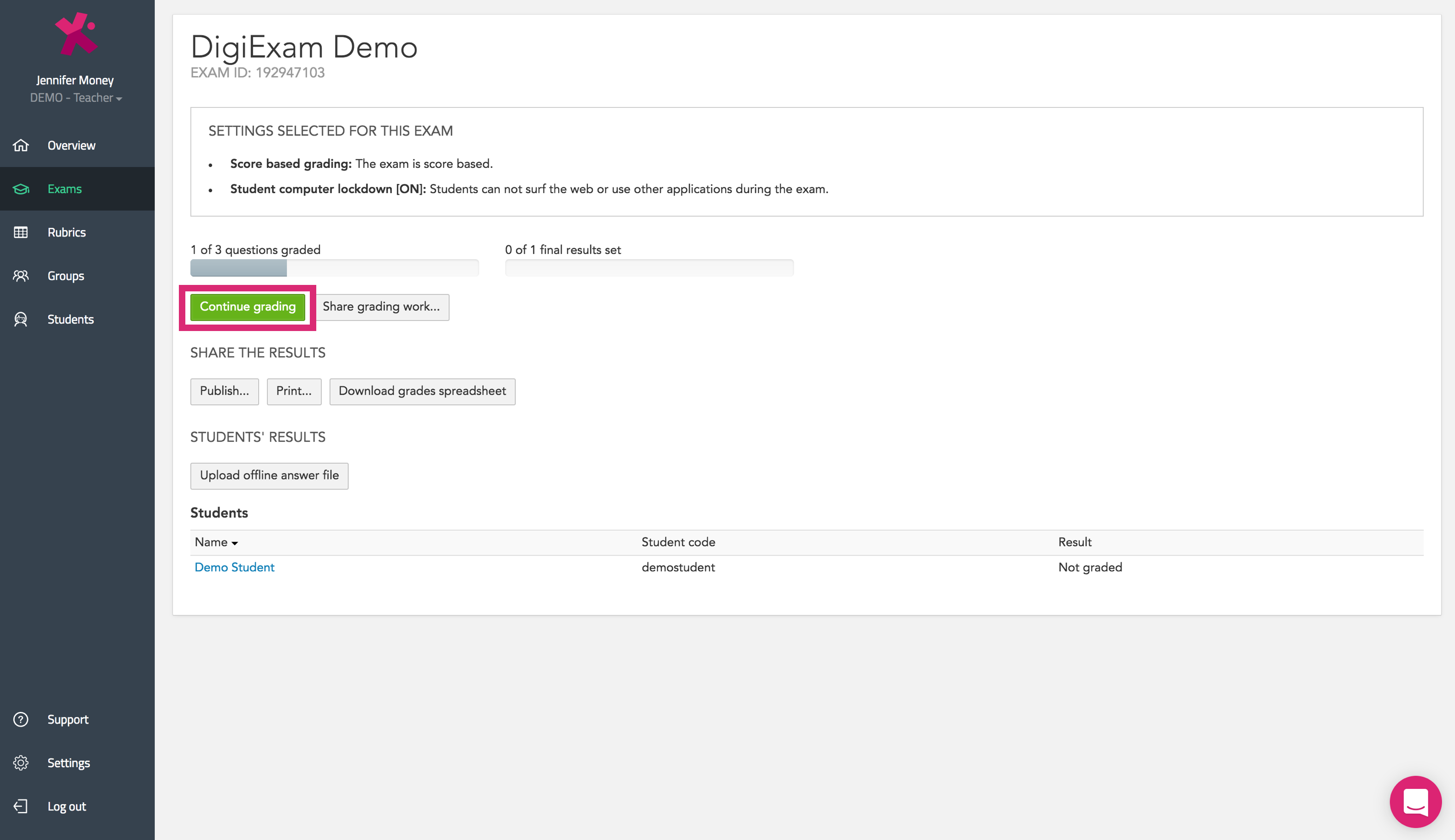Figure 3

Choose to grade by question or by student (see figure 4). The question and student you are currently viewing is highlighted in blue.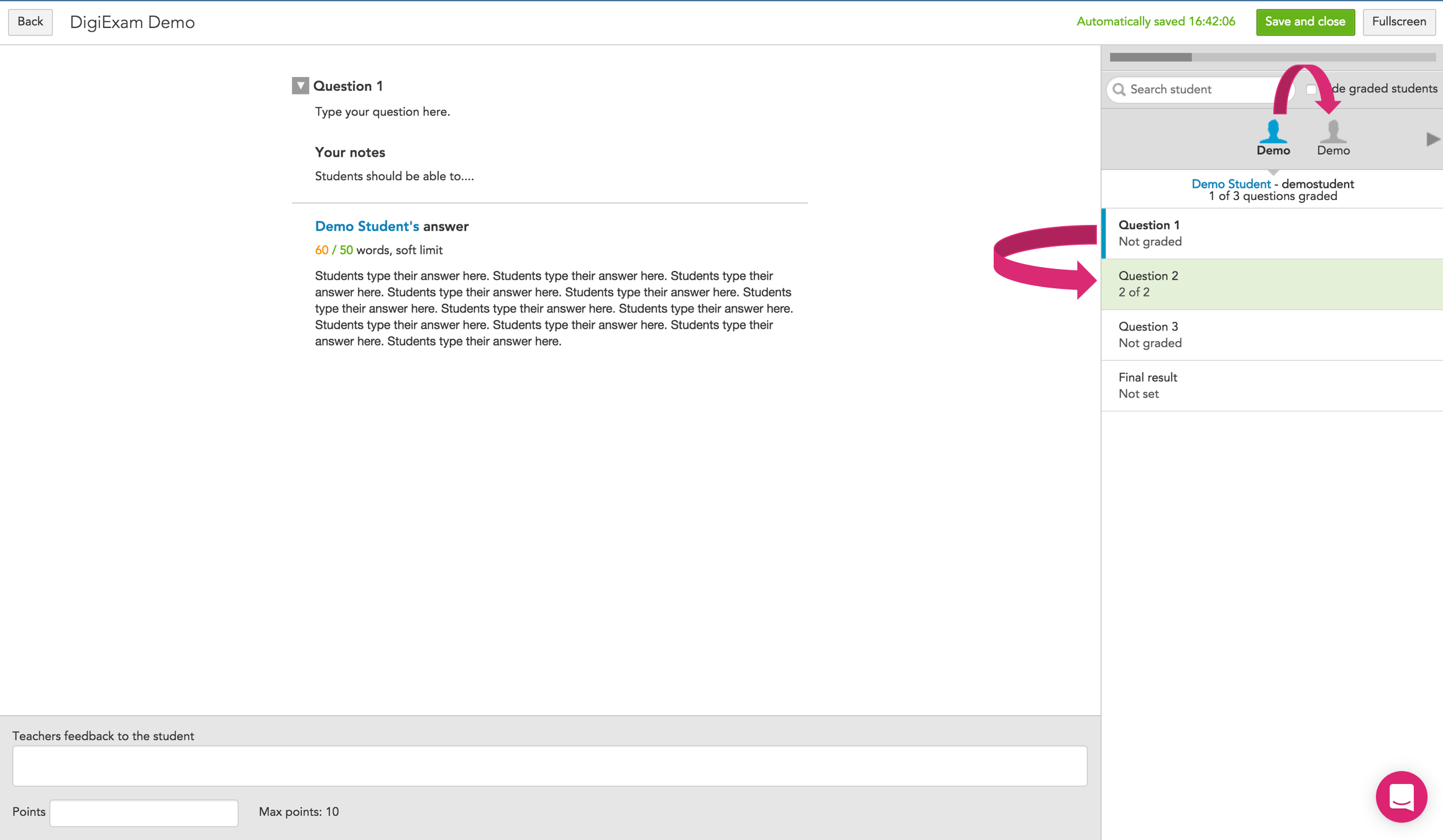Figure 4

### Text question

• Comment directly in the student’s answer by highlighting a portion of the student's response.  The annotation box will appear and you can comment or give points to the highlighted text (see figure 5).
• Provide additional comments and summarize your feedback in the box labelled Teacher’s feedback to the student (see figure 5).
• Enter the number of points earned in the Points box (see figure 5).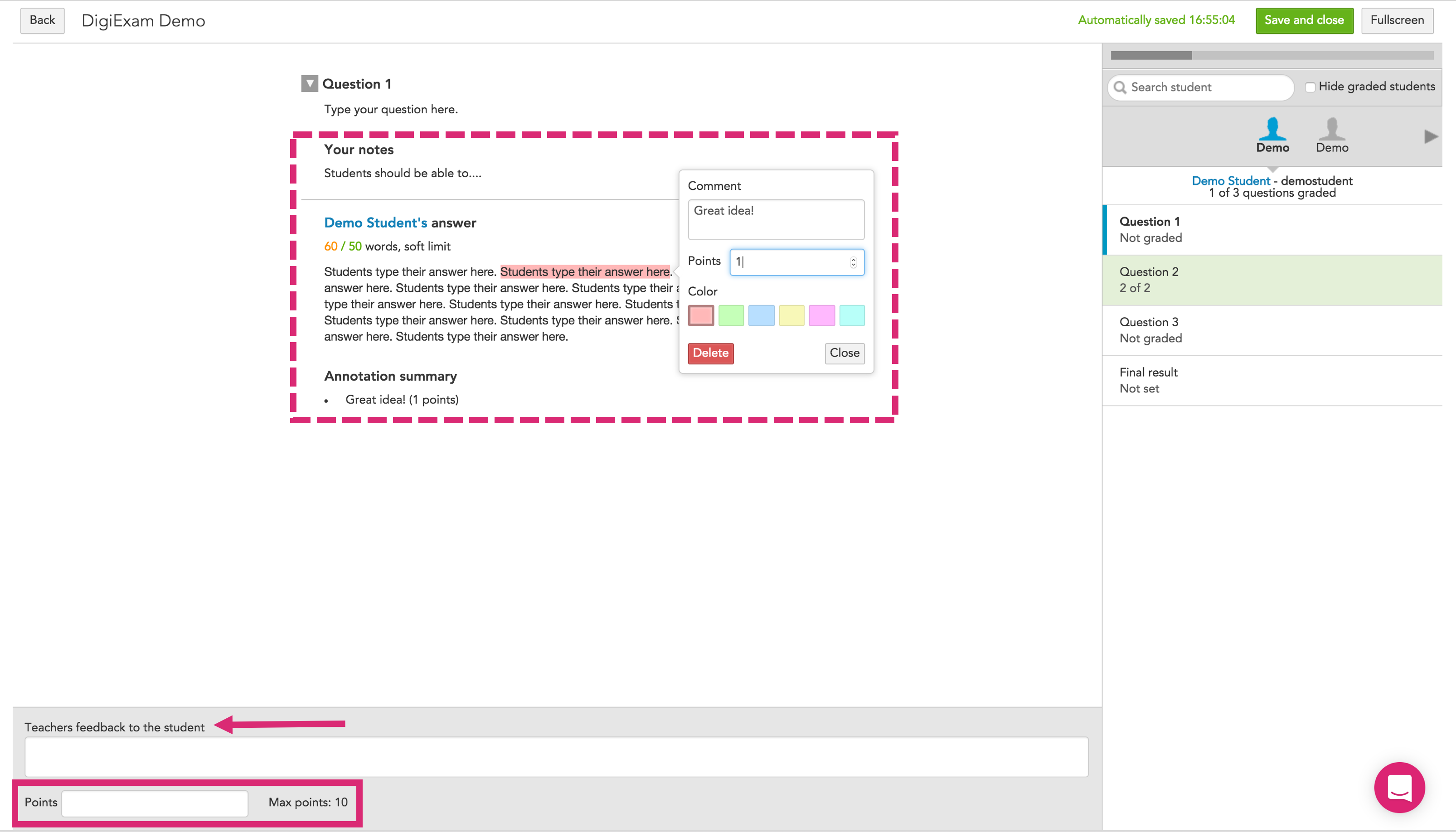Figure 5

### Multiple choice question

Automatically corrected and scored for you.

• You can provide comments in the box labelled Teacher’s feedback to the student (see figure 6).Figure 6

### Multiple response question

• Automatically corrected and scored.
• You can provide comments in the box labelled Teacher’s feedback to the student (see figure 7).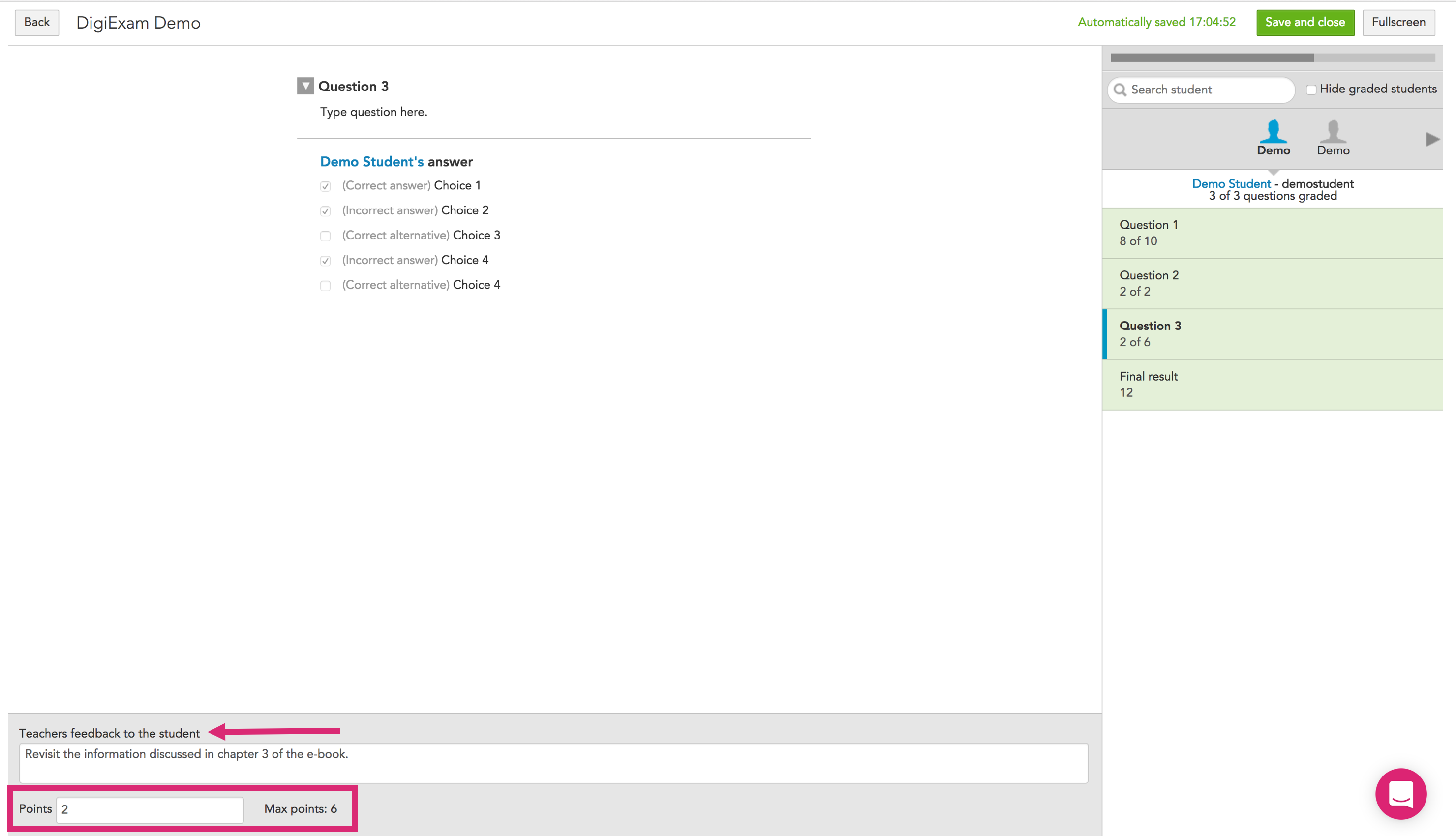Figure 7

### Word gap question

Word gap questions are graded automatically. This means that if an exam consists of word gap questions only, it will be moved to Graded right after it is ended.

The student can be graded as follows:

• Exact match: Student's response must be an exact match to the correct answer.
• Contains: Student's response must contain the correct answer.
• Case sensitive: Differentiate between capital and lower-case letters.

• All or nothing: The student must answer everything correctly to get max points. If the student miss to answer anything or answer something incorrectly, the student is awarded zero points
• Right minus wrong: The student gets plus points for every correct answer and minus point for each incorrect answer.
• Points per response: The student get plus points for each correct answer.

## Final result

• You can leave a final comment for the student in the box labelled Teacher’s final feedback to the student (see figure 8).
• Enter the final score earned.  You can enter the number of points (automatically calculated for you above) or you can enter a letter grade (see figure 8).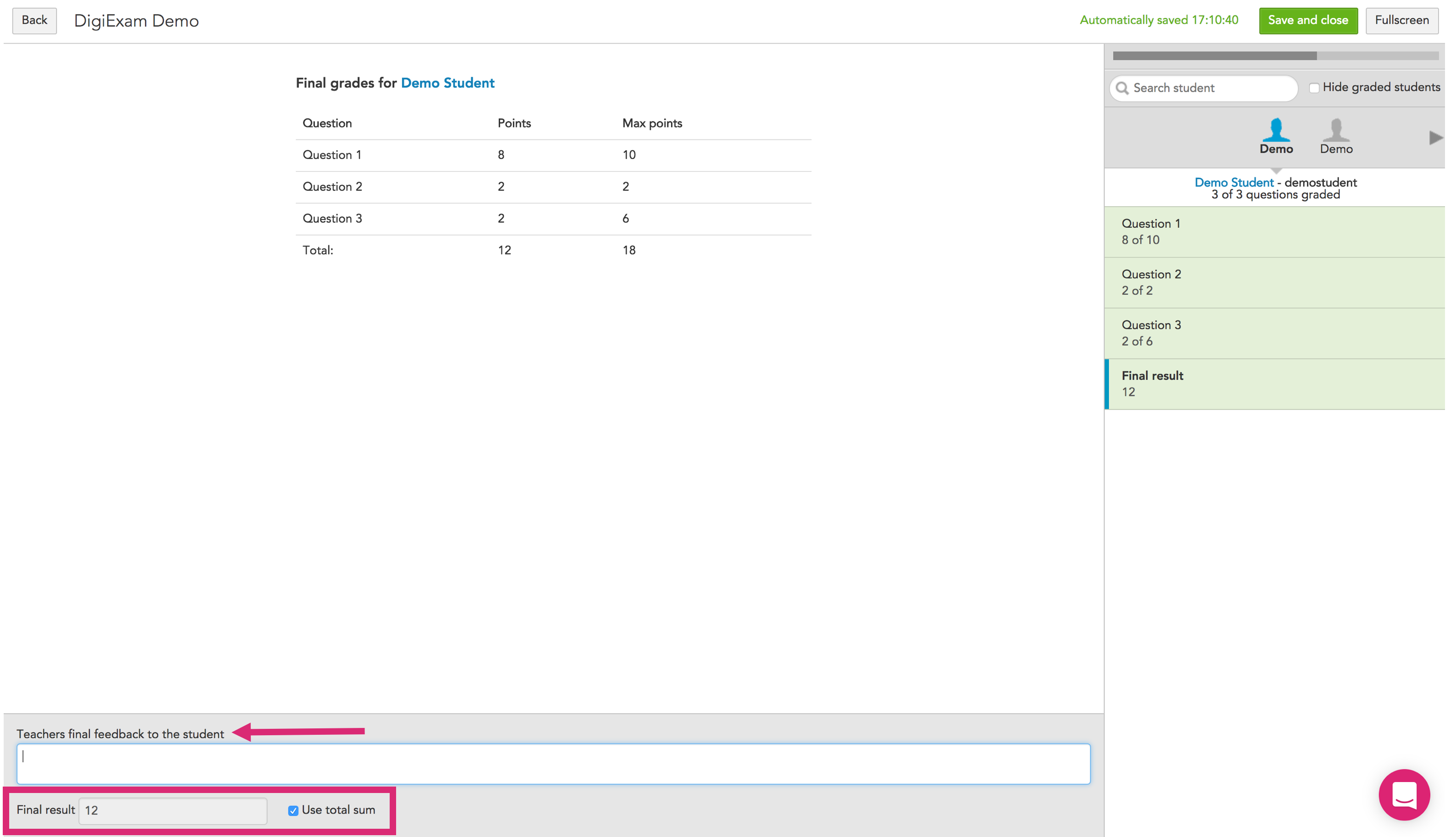Figure 8

Press the Back button in the top left corner to return to the grading overview (see figure 9).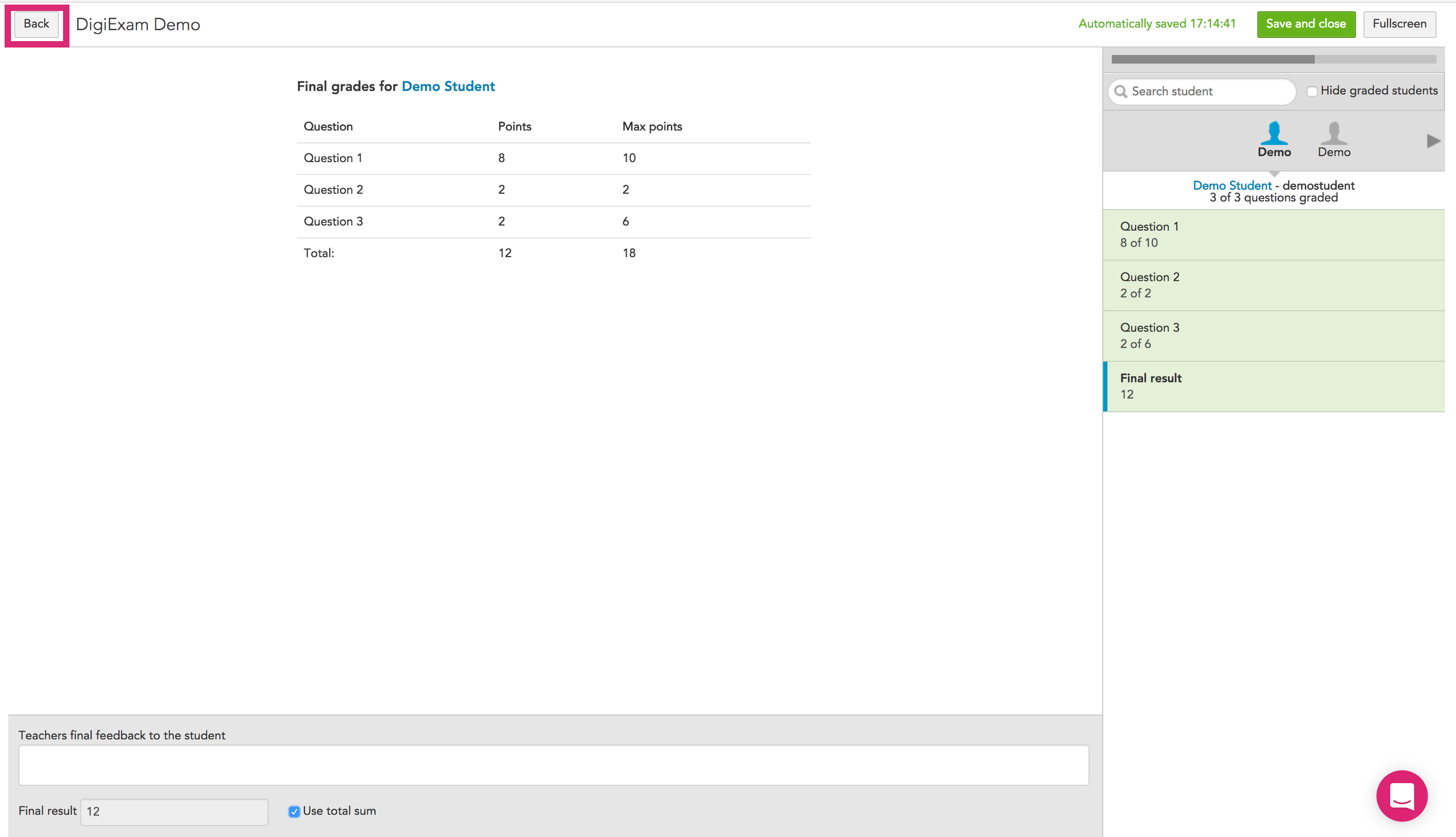Figure 9

Next article: Publish results and feedback